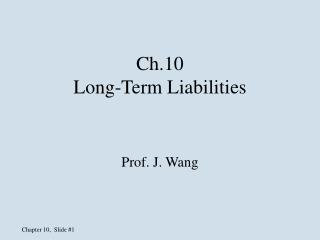DownloadDownload PresentationCh.10 Long-Term Liabilities

# Ch.10 Long-Term Liabilities

Télécharger la présentation## Ch.10 Long-Term Liabilities

- - - - - - - - - - - - - - - - - - - - - - - - - - - E N D - - - - - - - - - - - - - - - - - - - - - - - - - - -
##### Presentation Transcript

1. Ch.10Long-Term Liabilities Prof. J. Wang

2. 1 2 3 4 5 6 7 8 9 10 11 12 13 14 15 16 17 18 19 20 21 22 23 24 25 26 27 28 29 30 31 Balance Sheet Classification of Liabilities Current liabilities: Long-term liabilities: Due within one year of the balance sheet date Due beyond one year LO1

3. Long-Term Liabilities • Bonds payable • Notes payable • Leases • Deferred taxes • Pensions • Postretirement benefits

4. 1,000 \$10,000, 9% bond due 2019 Interest for Investor Borrower Bonds • Long-term borrowing arrangement • Interest paid at stated rate and times • Principal repaid at maturity date Investor Borrower LO2

5. Collateralized backed by specific assets in event of default Debentures backed only by general creditworthiness of issuer Bond Features

6. Term entire principal due on a specific single date Serial principal repaid in installments over time 1 2 3 4 5 6 7 8 9 10 11 12 13 14 15 16 17 18 19 20 21 22 23 24 25 26 27 28 29 30 31 Bond Features

7. Convertible into common stock Callable / Redeemable may be retired before maturity date Common Stock 1,000 Bond Features

8. Bonds Prices • Market rate v. stated rate • Market rate: interest rate offered by similar bonds in the market • Stated rate: interest offered by the specific bonds

9. On Jan. 1, 2006, Johnson Company issued \$10,000,000 bonds with a stated interest rate of 10%. The bonds mature in 20 years and interest is paid annually on Jan. 1.

10. Johnson promised two things: • Principal: \$10,000,000 paid at maturity • Interest: \$1,000,000 paid each year for 20 years (10,000,000x10%)

11. Calculating Bond Prices (1) Interest payments made each period PV = ? etc. \$\$ \$\$ \$\$ \$\$ (2) Principal due at maturity PV = ? \$\$\$\$\$

12. Investors earn the market interest rate (which is also referred to as the real/effective rate) regardless of the stated rate (which is also referred to as the nominal rate)

13. How much the bonds can be sold for depends on the market interest rate for similar bonds • Discount rate used in the present value calculations is the market interest rate

14. When stated rate is greater than the market rate, the bonds will sold at premium • When stated rate is less than the market rate, the bonds will sold at discount • When stated rate is equal to the market rate, the bonds will sold at face value

15. Compute the price of Johnson’s bonds assume the market interest rate is 12%

16. 2007 2008 2009 2006 Calculating Bond Prices (1) Interest payments (20 payments @ \$1,000,000) PV = ? \$1m \$1m \$1m \$1m (2) Principal of \$10,000,000 due at end of 2026 2026 \$10,000,000 PV = ?

17. Compute interest payment at stated rate (i.e., 10%)... …but discount @ market rate Example of Price Calculation • Present value: • Interest payments: • \$1,000,000 × 7.469 = \$7,469,000 • (PV; n = 20;i = 12%) • Principal payment: • \$10,000,000 × 0.104 = 1,040,000 • (PV; n = 20;i = 12%) • Bond issue price: \$8,509,000

18. Recording Bond Discounts Cash 8,509,000 Discount on Bonds Payable 1,491,000 Bonds Payable 10,000,000 To record the issuance of bonds payable. Assets = Liabilities + Owners’ Equity +8,509,000 –1,491,000 +10,000,000 LO4

19. Balance Sheet Presentation of Bond Discount Long-term liabilities: Bonds payable \$10,000,000 Less: Discount on bonds payable 1,491,000 \$ 8,509,000

20. Determining Bond Prices • Compute the price of Johnson’s bonds assume the market interest rate is 8%

21. Compute interest payment at stated rate (i.e., 10%)... …but discount @ market rate Example of Price Calculation • Present value: • Interest payments: • \$1,000,000 × 9.818 = \$9,818,000 • (PV; n = 20;i = 8%) • Principal payment: • \$10,000,000 × 0.215 = 2,150,000 • (PV; n = 20;i = 8%) • Bond issue price: \$11,968,000

22. Recording Bond Premiums Cash 11,968,000 Bonds Payable 10,000,000 Premium on Bonds Payable 1,968,000 To record the issuance of bonds payable. Assets = Liabilities + Owners’ Equity +11,968,000 +10,000,000 +1,968,000

23. Balance Sheet Presentation of Bond Premium Long-term liabilities: Bonds payable \$10,000,000 Plus: Premium on bonds payable 1,968,000 \$11,968,000

24. Bonds Sold at Face Value Cash 10,000 Bonds Payable 10,000 To record the issuance of bonds at face value. Face value of bonds = Sales price When stated rate=market rate

25. Interest Rates and Bond Prices BONDS ISSUED: IF STATED RATE: Above face value (at a premium) At face value Below face value (at a discount) > MARKET RATE = MARKET RATE < MARKET RATE

26. Amortization of Bond Premiums and Discounts Transferring an amount from the discount or premium account to interest expense over the life of the bond using the effective interest method Premium reduces interest expense Discount increases interest expense LO5

27. When bonds are issued at discount: Interest expense = (cash interest) + (discount amortization) = \$1,000,000 + (1,491,000/20) = \$1,074,550 12/31/06 Dr. Interest Expense 1,074,550 Cr. Interest payable 1,000,000 Cr. Discount on B/P 74,500 1/1/07 Dr. Interest payable 1,000,000 Cr. Cash 1,000,000

28. Total interest expense during the life of the bonds = 1,000,000*20 + 1,491,000 = \$21,491,000

29. Prepare appropriate journal entries for Johnson Company when the bonds were issued at premium